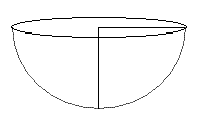Home > Geometry calculators > Volume of a Hemi-Sphere calculator

Volume -
Volume - Hemi-SphereFormula : Curved Surface Area (CSA) = 2 pi r^2Total Surface Area (TSA) = 3 pi r^2Volume (V) = 2/3 pi r^3diameter (d) = 2r

6. I know that for a Hemi-Sphere = . From this find out of the Hemi-Sphere.
pi =
Decimal Place =

 SolutionExampleVolume FormulaAll Problems